# Overview

This processor allows to configure a Machine Learning model and build a  pipeline to train then save this model.

`Note: Changed machine learning algorithm implementations in Spark 3 may slightly change results compared to Spark 2.`

# Input

The processor has two input nodes:

• Left Node: contains the training Data.
• Right Node: contains the test/forecast Data.
Note that: the two input sets must match in the schema (Column Names and types) otherwise a Processor Validation Error is generated

To make sure that the training and test datasets have the same schema, the Horizontal Split processor can be used.

Important: If the model is trained to classify, the dependent column has to be of type string. If it is trained for regression, the dependent column has to be of type numeric.

# Configuration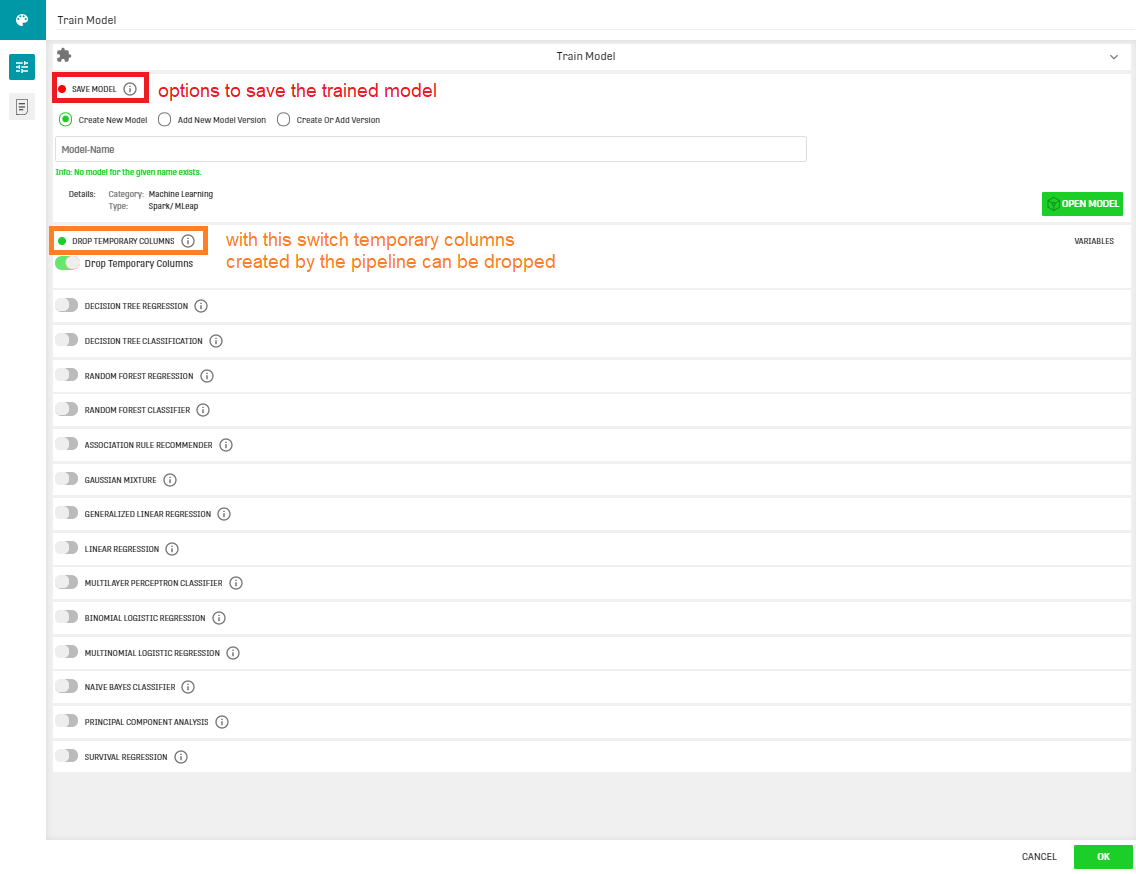The main purpose of this processor is to train a Machine Learning model and save it. Therefore, the Save Model configuration field is mandatory and offers three possibilities to save the trained model:

•  Create New Model: a model is a generic resource so it must have a unique name across a ONE DATA domain, when provided a name of an existing model, a warning is shown.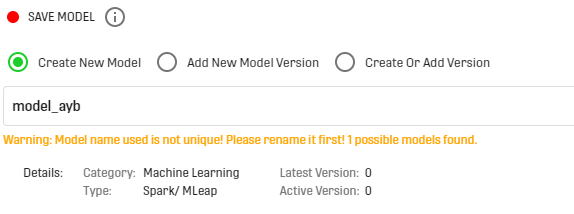• Add New Model Version: an existing model should be provided, otherwise, a warning is shown.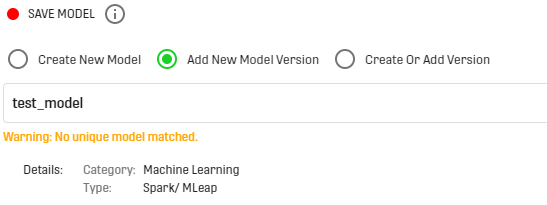• Create Or Add Version: if an existing model is provided, then the processor will increase the selected model's version otherwise it will create the model.

## Further Configurations

NOTE THAT: at least one model configuration has to be provided, otherwise the processor will generate a Processor Validation Error

• Association Rule Recommender: provides recommendations based on Association Rules. The configuration of this model is as follows: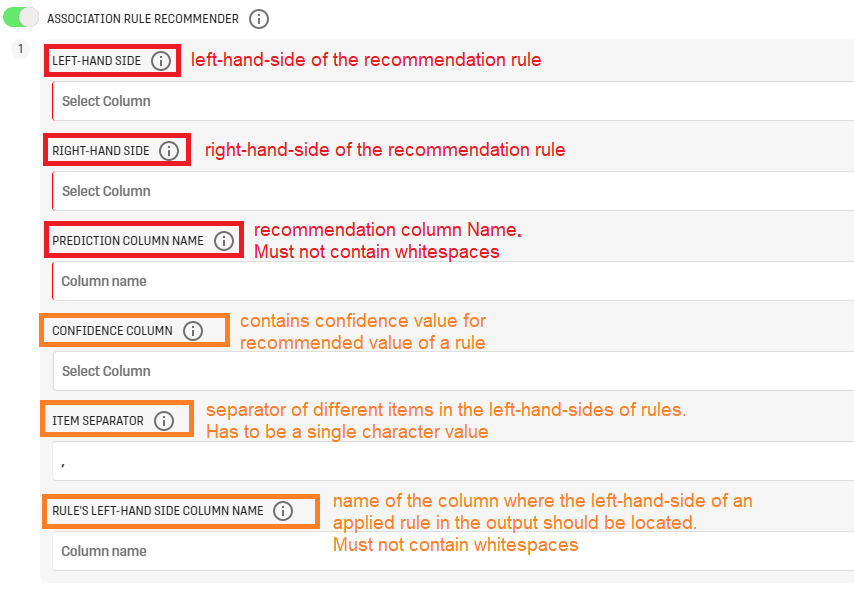Further documentation about processors related to association rules and recommendation can be found in the related articles section.

• Gaussian Mixture: used to provide the probabilistic Gaussian Mixture of the input Dataset. It can be configured as follows: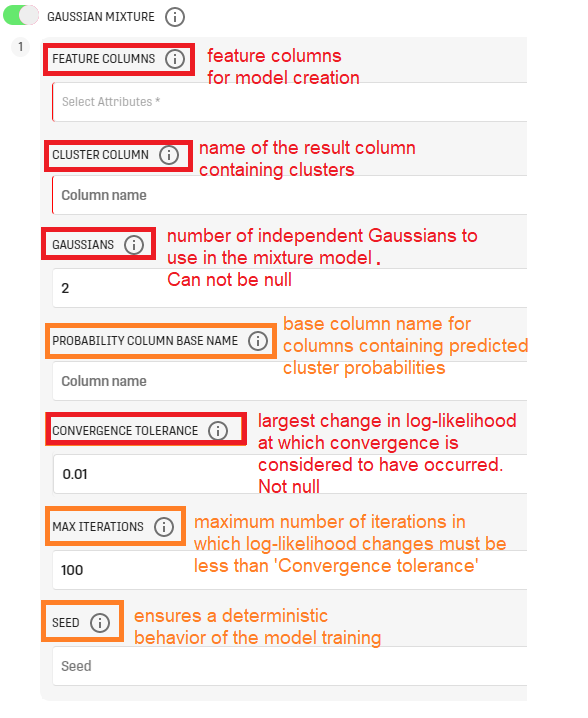The GLM generalizes linear regression by allowing the linear model to be related to the response variable via a link function and by allowing the magnitude of the variance of each measurement to be a function of its predicted value.

The configuration menu looks as follows: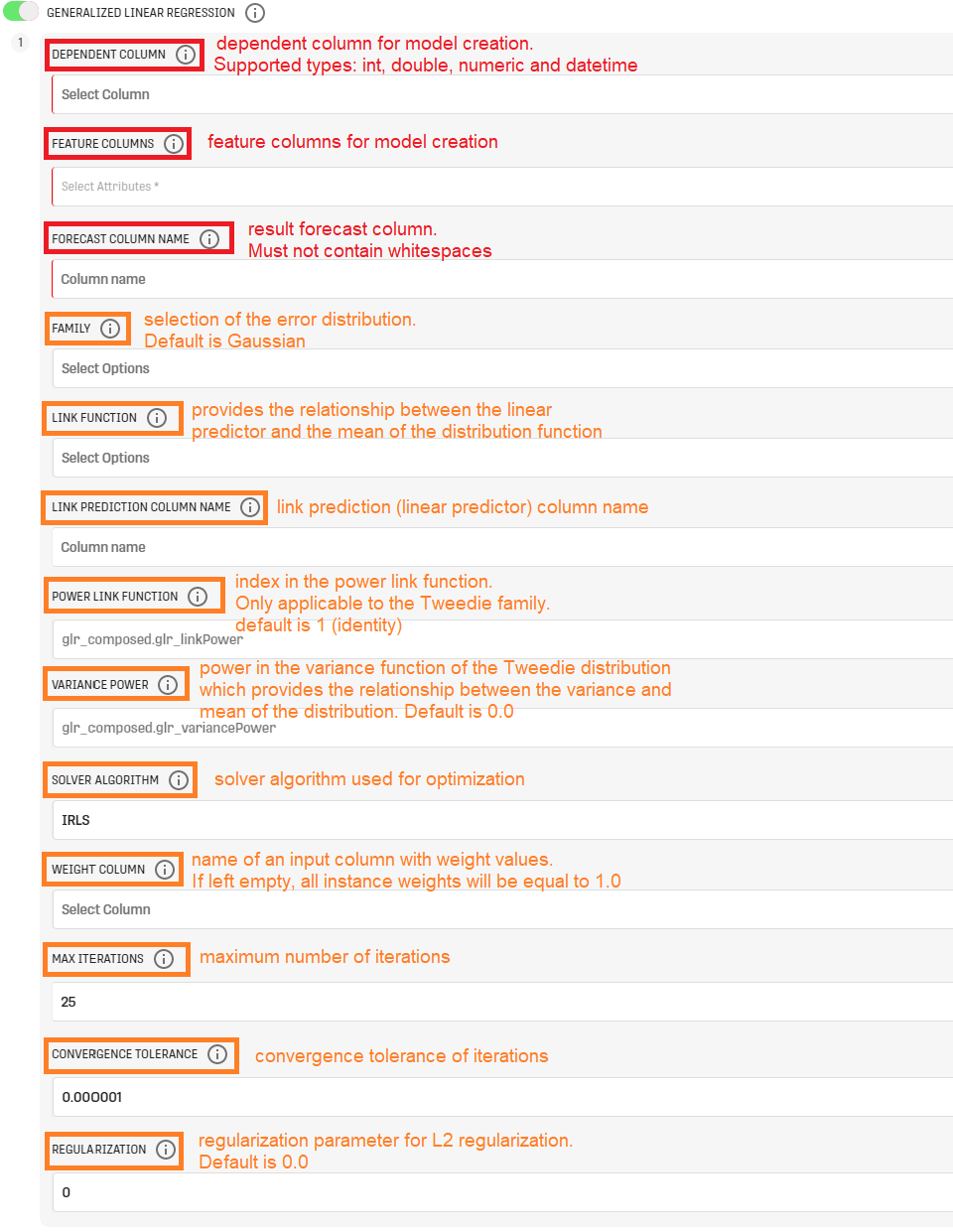• Linear Regression: allows to create a Linear Regression model to study the relationship between a dependent variable and a set of independent variables. It can be configured as follows: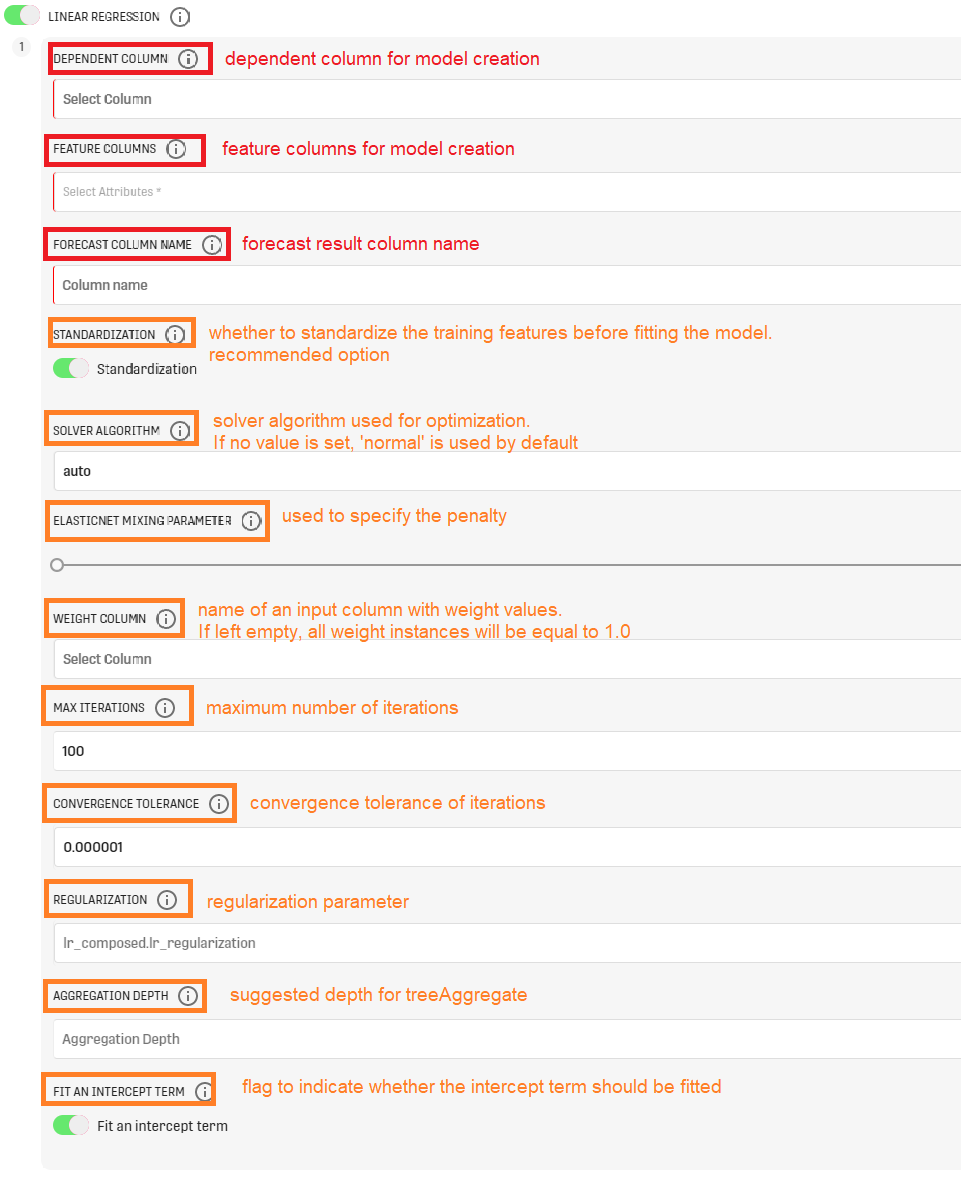• Multilayer Perceptron Classifier: creates a Multilayer Perceptron model out of the input Dataset. The configuration is the following: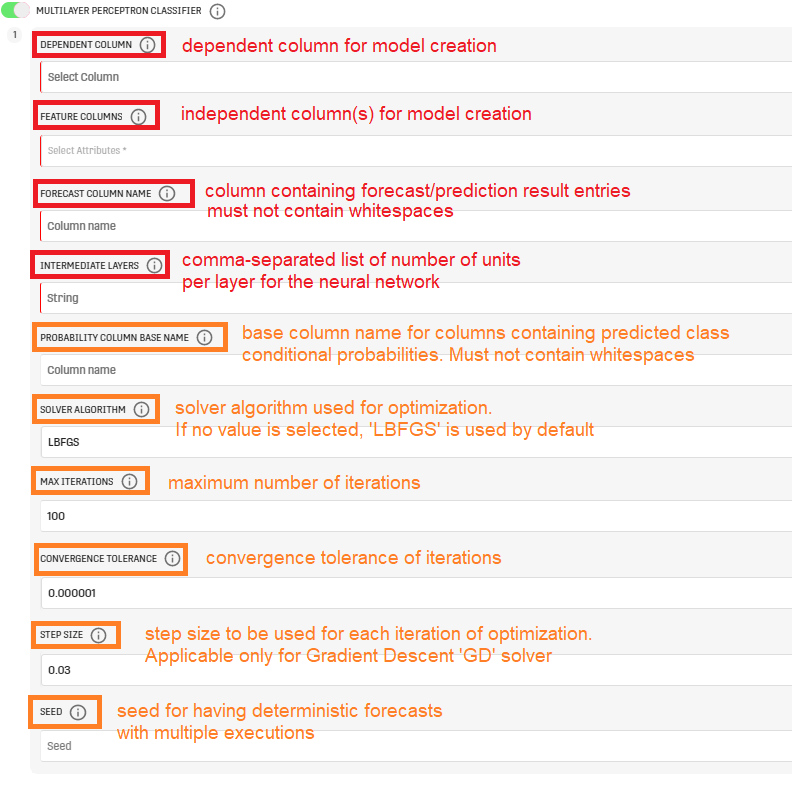• Binomial Logistic Regression: creates a Binomial Regression model to study the relationship between a binary dependent column and a set of independent columns. The configuration menu is the following: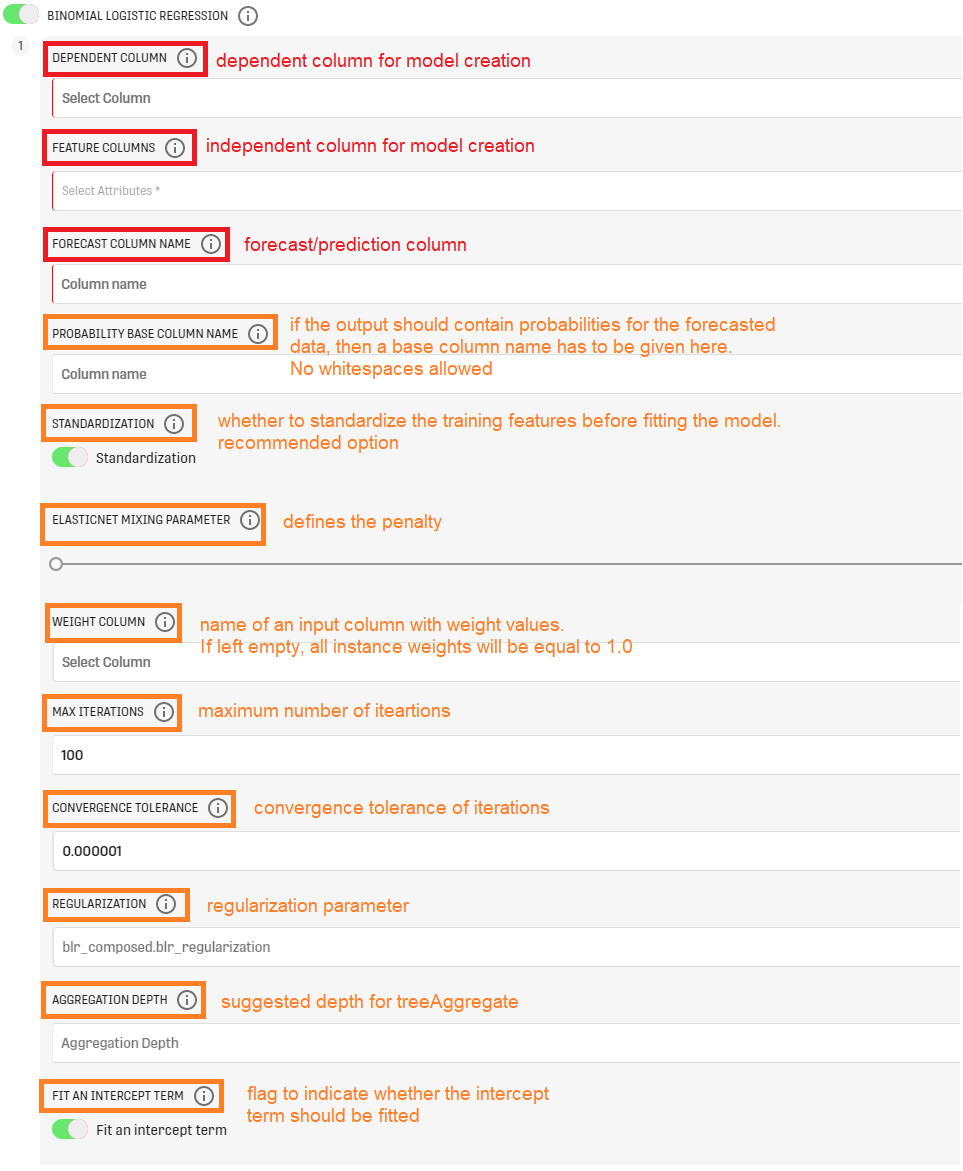• Multinomial Logistic Regression: very similar to Binomial Logistic Regression. In a Multinomial Logistic Regression the dependent column can have more than two classes. The configuration interface is similar to the configuration of binomial logistic regression.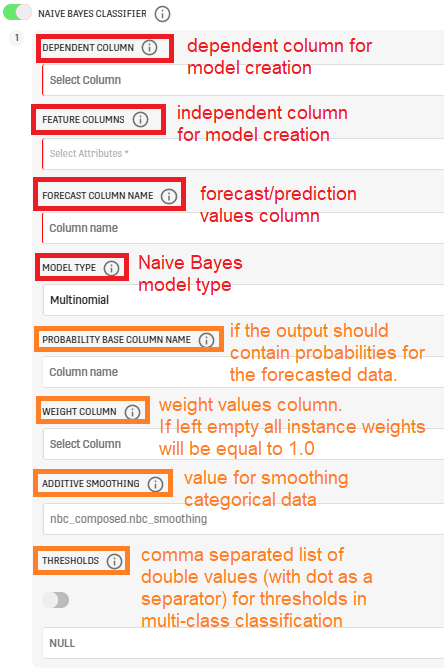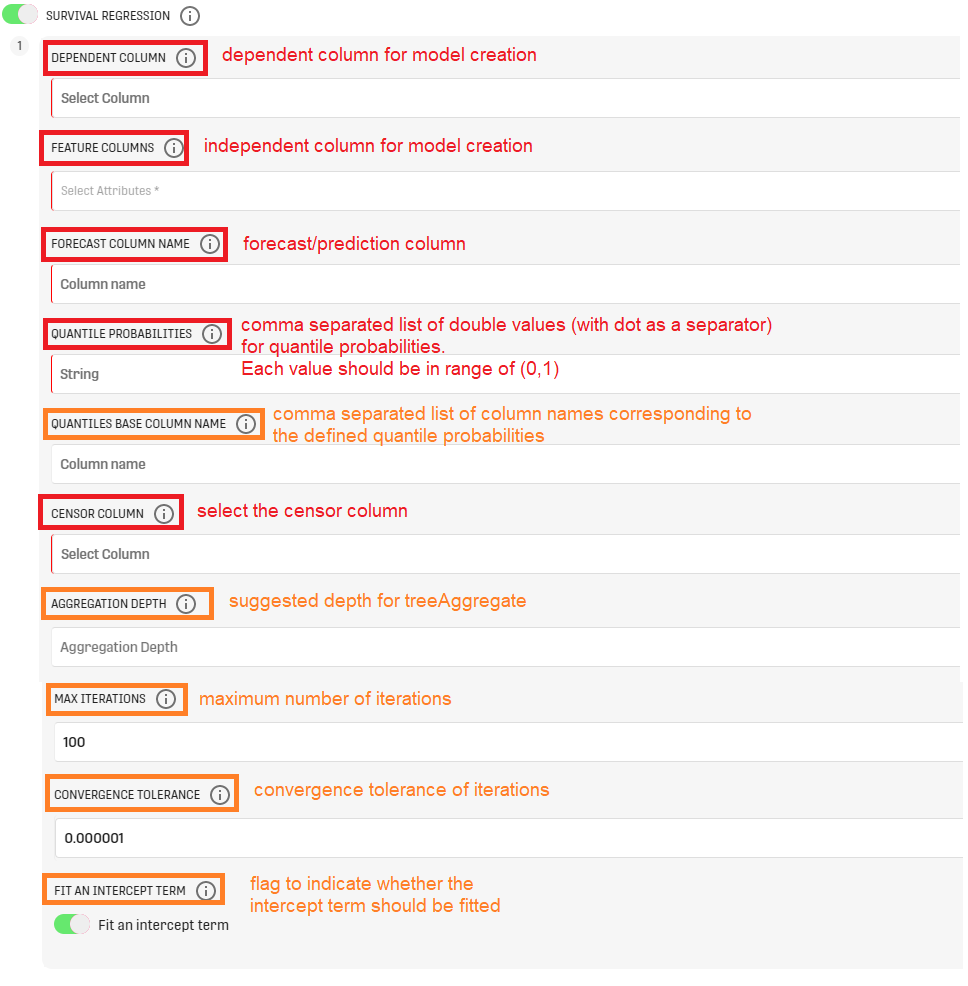NOTE THAT: It is possible to create multiple groups within the same configuration option using the button "Add Group". It is also possible to configure multiple options, but at least one valid configuration has to be provided

# Output

The Processor returns a result table with the input data and the forecasted values. Withing the processor, a JSON object including descriptive details of the saved model is also returned.

Furthermore, a new model/version will be created according to the specified configuration.

In case the model is trained using a numerical dependent column, the Forecast Metrics Processor can be used to evaluate the performance of the trained model. In case of categorical Data, the Distinct Rows Processor can be configured to extract distinct entries from the result table using the dependent and prediction columns. Then the Row Count Processor can be used to count the total wrong predictions.

# Example

## First Example

In this example a Decision Tree Classification model will be trained on two similar datasets provided by two Custom Input Table processors: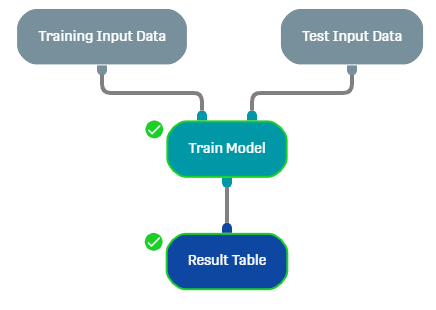The input training data is the following: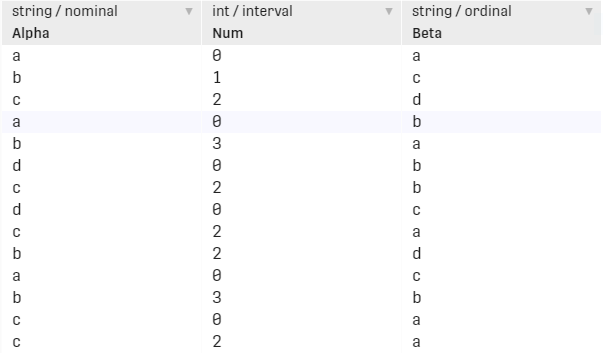The input test data is the following: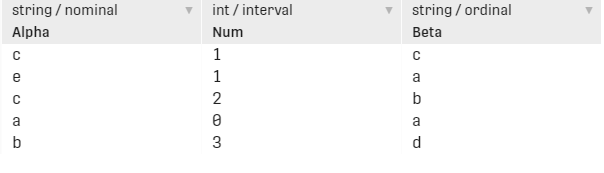The configuration is the following: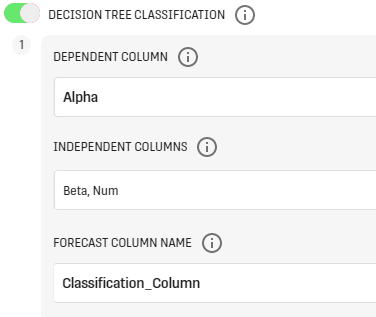### Results

#### Json Object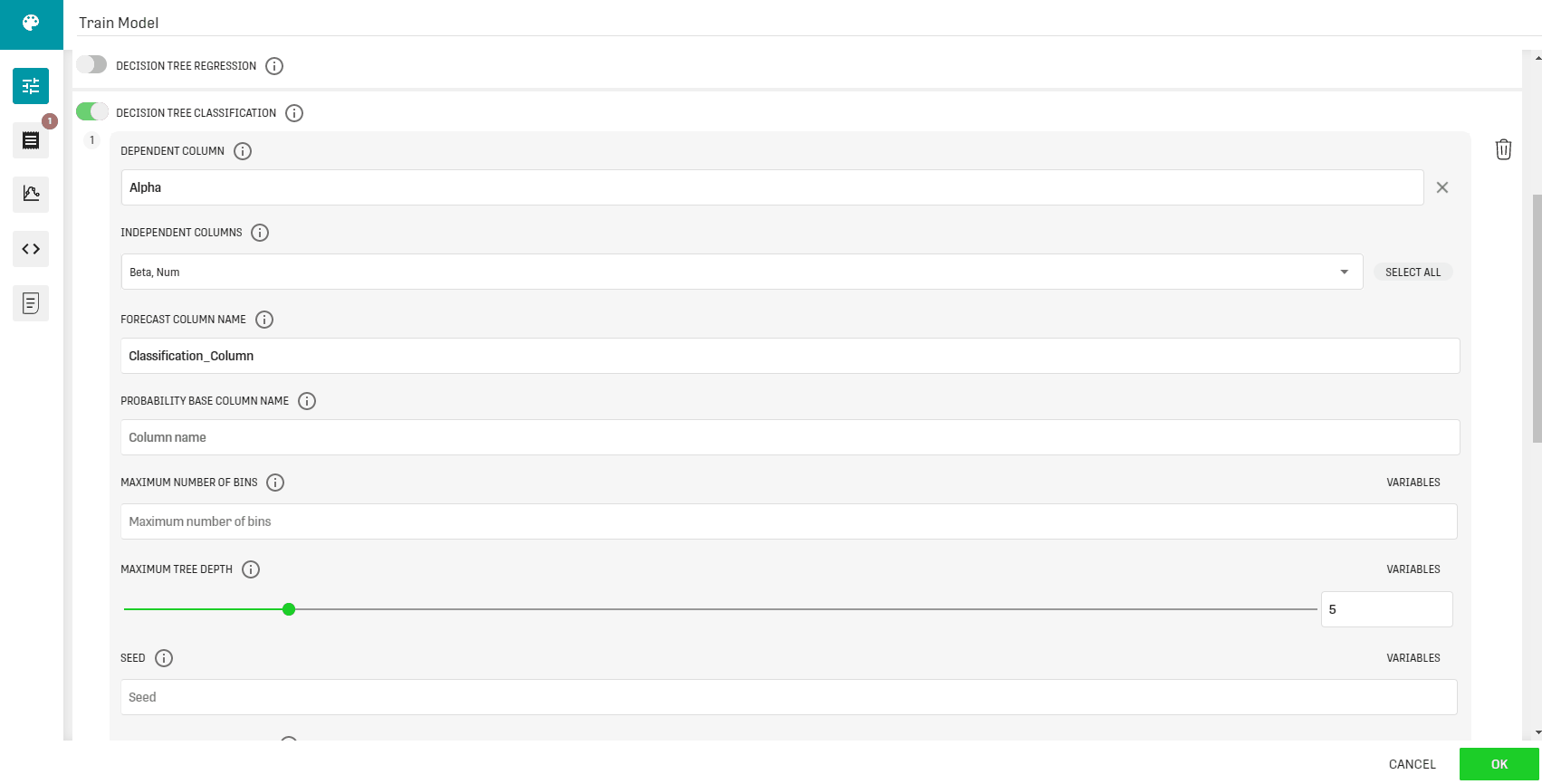#### Model predictions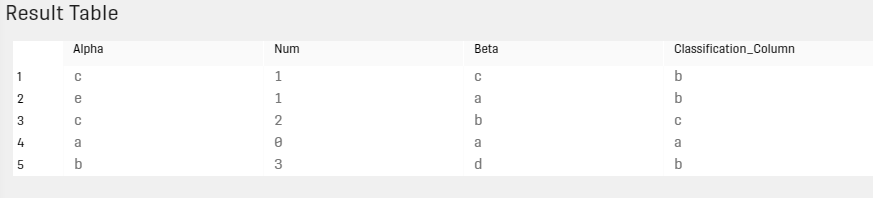## Second Example

In this example the Train Model processor was used to instantiate and train a Multilayer Perceptron on the Iris Dataset. The Dataset is loaded using the Data Table Load Processor and split into training and test datasets using the Horizontal Split Processor.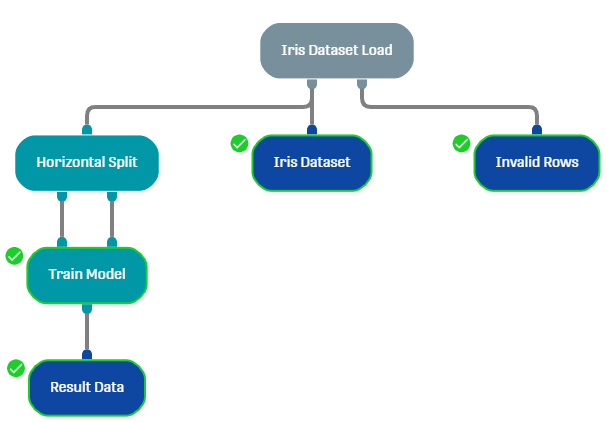The model is configured as follows: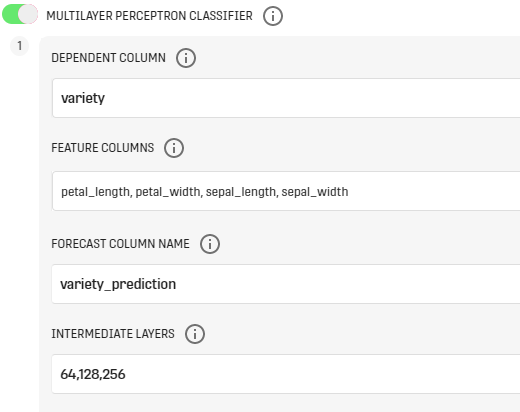In this example, we created a three layer MLP (aka Artificial Neural Network) where the number of units per hidden layer was 64, 128 and 256 respectively.

### Results

#### Json Object#### Model predictions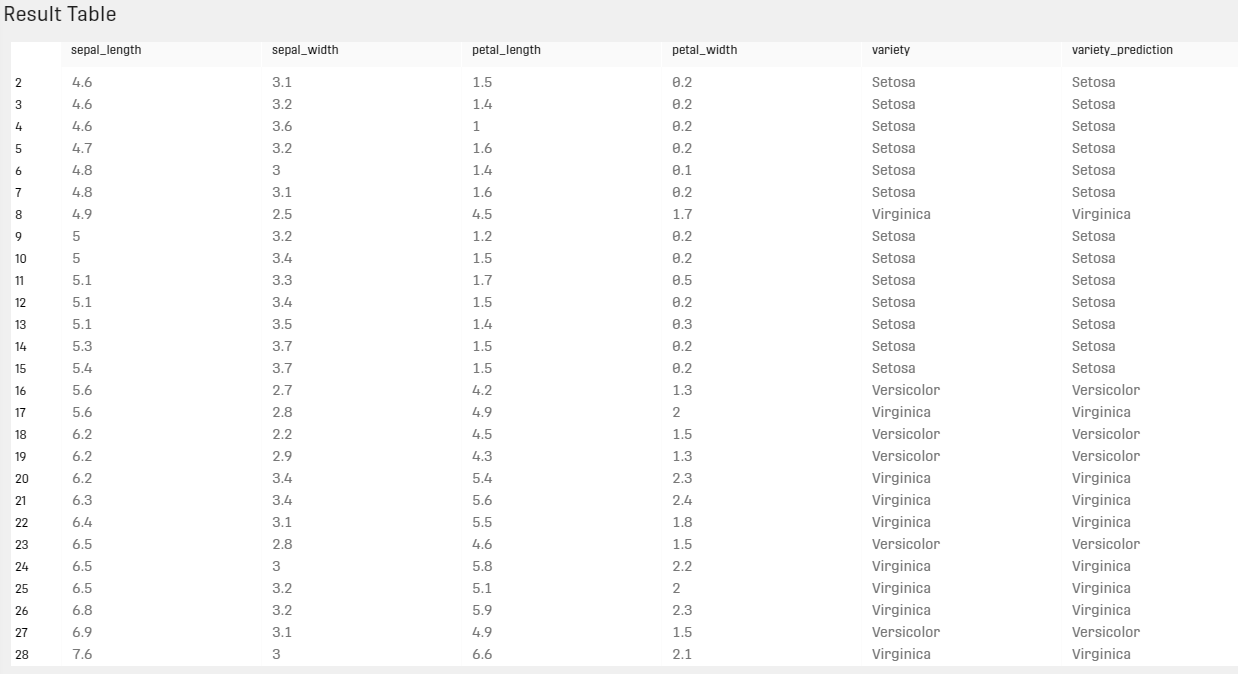# Related Articles

Association Rule Generation Processor

Association Rules Application Processor

ALS Recommender Processor

Grouped Forecast Processor

Forecast Metrics Processor